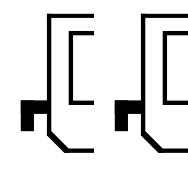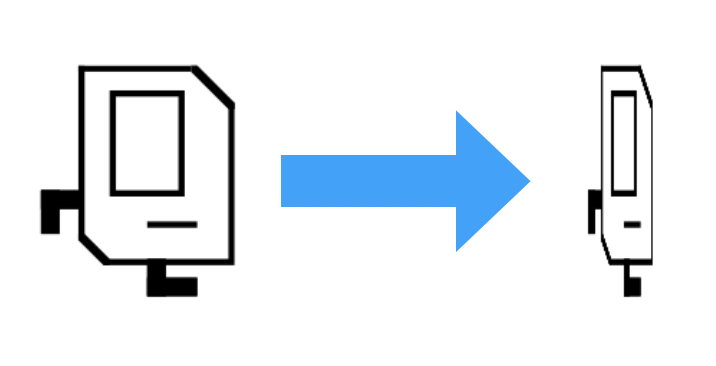# Section #3: Parameters, Return Values, Lists & Images

October 4th, 2020

Written by Brahm Capoor, Juliette Woodrow, Parth Sarin, Kara Eng, Tori Qiu and Peter Maldonado

This week in section, your goal is to practice and gain familiarity with the principles of parameters and return values, experiment with lists, and work on some interesting image problems using the SimpleImage library. We'll also explore some interesting applications of doctests in the debugging process.

## Parameters Review

### Checking Ranges

Implement the following function which takes in 3 integers as parameters:

        
def in_range(n, low, high)
"""
Returns True if n is between low and high, inclusive.
high is guaranteed to be greater than low.
"""



Next, complete the program by writing a main function which reads in three integers from the user and calls in_range to determine whether or not the second number is in between the first and third. Here are a few sample runs of the program (user input is italicized):

        
Enter first number: 42
Enter second number: 8
Enter third number: 50
8 is not in between 42 and 50

Enter first number: 8
Enter second number: 42
Enter third number: 50
42 is in between 8 and 50

Enter first number: 50
Enter second number: 42
Enter third number: 8
42 is in between 50 and 8



### FizzBuzz

In the game Fizz Buzz, players take turns counting up from one. If a player’s turn lands on a number that’s divisible by 3, she should say “fizz” instead of the number, and if it lands on a number that’s divisible by 5, she should say “buzz” instead of the number. If the number is both a multiple of 3 and of 5, she should say "fizzbuzz" instead of the number. A spectator sport, it is not.

What it is, however, is an interesting problem in control flow and parameter usage. Write a function called fizzbuzz which accepts as a parameter an integer called n. The function should count up until and including n, fizzing and buzzing the correct numbers along the way. Once it's done, the function should return how many numbers were fizzed or buzzed along the way.

Next, complete your program by writing a main function that reads in an integer from the user and plays fizzbuzz until it counts to the number. Here's a sample run of the program (user input is italicized):

          
Number to count to: 17
1
2
Fizz
4
Buzz
Fizz
7
8
Fizz
Buzz
11
Fizz
13
14
Fizzbuzz
16
17



### Medical Test Simulator

A team of doctors and scientists has developed a new medical test for a rare disease that they say is 99% accurate. However, they warn, that number is deceiving. Your job is to write a program that confirms this.

To be precise about our wording, a test being 99% accurate means that for every 100 people it tests, 99 people will be correctly diagnosed as sick or healthy.

To explore the implications of this, suppose we have a population of 10000 people, of whom 1%, or 100 people, have the disease. Your job is to write a function called simulate_tests, which predicts each of 4 quantities:

• The number of true positives: the number of sick people who are correctly diagnosed as sick.
• The number of false positives: the number of healthy people who are incorrectly diagnosed as sick.
• The number of false negatives: the number of sick people who are incorrectly diagnosed as healthy.
• The number of true negatives: the number of healthy people who are correctly diagnosed as healthy.

In order to be able to generalize this prediction to other diseases and contexts, your function should accept the following parameters:

        
def simulate_tests(num_people, test_accuracy, infection_rate)
"""
num_people: the number of people getting the test
test_accuracy: how accurate the test is
infection_rate: the proportion of the population with the disease
"""



In the specific example discussed above, the function would be called with these parameters:

        
simulate_tests(10000, 0.99, 0.01)



Your function should print all 4 quantities mentioned above and return the fraction of incorrect positive results: that is, the number of incorrect positive results divided by the total number of positive results.

This is a tricky process, so we'll break it down into steps. To simulate a single person's test, take these steps:

1. Randomly choose whether the person is sick with a probability of 0.01.
2. Randomly choose whether the test is correct for them with a probability of 0.99.

You might wish to make use of the following piece of code, which evaluates to True with probability prob

        
is_true = random.random() < prob



random.random() returns a random number between 0 and 1, whose probability of being less than prob is prob, provided that prob is between 0 and 1. For example, a random number between 0 and 1 has a 50% chance of being less than 0.5, and so to get a variable that is True with 50% probability, you'd use the following line:

              
is_true = random.random() < 0.5



Once you've written this function, complete the program by writing a main function that reads in a number of people, a test accuracy rate and an infection rate from the user, calls simulate_test and then prints the proportion of incorrect positive results (which simulate_test should return). You may assume the user types in valid inputs for each of these values, although you should decide what type they should be.

Here's some sample output of the program (user input is italicized):

        
Number of people: 10000
Test accuracy: 0.99
Infection rate: 0.01
True positives: 93
False positives: 113
False negatives: 1
True negatives: 9793
54.85436893203883% of positive tests were incorrect



As you can see, the results are pretty surprising: nearly 55% of people told they had the disease didn't actually have it. Intuitively, why might this be? Is our result just anomalous?

## Lists Review

### Collapse

Implement the following function:

        
def collapse(lst):
"""
Accepts a list of integers as a parameter and returns a new
list containing the result of replacing each pair of integers
with the sum of that pair.

If the list stores an odd number of elements, the final element
is not collapsed

>>> nums = [7, 2, 8, 9, 4, 13, 7, 1, 9, 10]
>>> collapse(nums)
[9, 17, 17, 8, 19]
>>> nums = [1, 2, 3, 4, 5]
>>> collapse(nums)
[3, 7, 5]
"""



### Distinct Elements

Implement the following function:

        
def distinct_elements(lst):
"""
Returns a list of all the disinct elements in lst, in the order that
they first occur in lst.
>>> distinct_elements([1, 2, 3, 4])
[1, 2, 3, 4]
>>> distinct_elements([1, 1, 2, 2, 3])
[1, 2, 3]
>>> distinct_elements(['hello', 'hello', 'hello', 'hello', 'hello'])
['hello']
>>> distinct_elements([])
[]
"""



### Rotate

Implement the following function:

        
def rotate_list_right(lst, n):
"""
returns a 'rotate' version of the list that rotates numbers
to the right n times. Each element in numbers is shifted
forward n places, and the last n elements are moved to
the start of the list.

Your function should not change the list that is passed as a
parameter.

>>> rotate_list_right([1, 2, 3, 4, 5], 2)
[4, 5, 1, 2, 3]
"""



## Images

Implement the following functions to familiarize yourself with the various ways you can work with images. Each function takes in a string filename, which can then be converted into a SimpleImage. Each function should end by displaying the image, which can be done by calling the .show() function on an image.

### Double Left

            
def double_left(filename):
"""
Takes the left half of image, and copies it on top of the right half.
"""### Squeeze Width

                  
def squeeze_width(filename, n):
"""
A funhouse mirror effect governed by the int parameter n.
Create a new image with the same height as the original, but
with a width that is n times smaller than the original's.
Copy the original image such that it is squeezed horizontally.

For example, if n = 4, the pixels in the output image with an
x coordinate of 0 would be copies of the input pixels with
an x coordinate of 0 * 4 = 0, while pixels in the output
image with an x coordinate of 1 would be copies of the input
pixels with an x coordinate of 1 * 4 = 4, and so on.
"""### Crop Image

            
def crop_image(filename, n):
"""
crops the image to fit inside of a frame by shaving
n pixels from each side of the image.

You may assume that n is less than half the width of
the image.
"""## Debugging with Doctests

In this problem, we'll work through using doctests to help us debug a program. Suppose we're trying to write a function which given two integers, calculates the Greatest Common Divisor of both numbers. The Greatest Common Divisor of two numbers is the largest number that is a factor in both of them. In greatest_common_divisor.py, we've provided the following buggy implementation of a function called greatest_common_divisor:

        
def greatest_common_divisor(a, b):
"""
Return the greatest common divisor of a and b.

>>> greatest_common_divisor(4, 16)
4
>>> greatest_common_divisor(16, 4)
4
>>> greatest_common_divisor(9, 24)
3
"""

lower = a

gcd = 1
for i in range(1, lower):
if a % i == 0 or b % i == 0:
gcd = i



At first glance, this looks like a reasonable way of solving the problem, but it turns out there are several issues. By running the doctests for the function, try identifying what the bugs are and correcting them. You might wish to add more doctests to more exhaustively test your function.